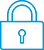cancel
Showing results for
Did you mean:It has been a while since anyone has replied. Simply ask a new question if you would like to start the discussion again.Level 1
10 5 0 0
Message 1 of 2
419
Flag Post

Solved!

# How to solve for unknown constants such as F(x)=ax^3+bx^2+cx+d when given coordinates on t HP PrimeHP Recommended
HP prime

How to solve for unknown constants such as F(x)=ax^3+bx^2+cx+d when given coordinates on HP Prime

for example,

the cubic F(x)=ax^3+bx^2+cx+d

has a turning point at x=-2 and x=4

has a y-tercept at -3

find the values of (a,b,c,d)

Tags (1)
1 ACCEPTED SOLUTION

Accepted SolutionsLevel 8
650 635 124 222
Message 2 of 2
Flag PostHP Recommended

Until somebody replies with a more efficient method, here's one way to attack that problem on a Prime.

If the turning points are at -2 and 4, then the derivative of the function must equal zero when x=-2 and when x=4. If the derivative of the function isn't obvious, Prime can yield it by typing a single quote mark after it.

Now substitute -2 and 4 for x in the derivative, simplify, and set the result equal to zero. This yields these two equations:

12a-4b+c=0

48a+8b+c=0

Use CAS to solve the system, like this:

solve( [ 12*a-4*b+c=0, 48*a+8*b+c=0 ], [a, b, c])

This yields the general solution [ a=-1/24*c, b=1/8*c, c=c ]

Therefore an infinite number of solutions exist.  The most obvious one is obtained by multiplying these equivalents by -24:

a=1, b=-3, c=-24

The problem also specified that the y-intercept is -3, which of course simply means that d=-3.

Therefore one correct answer is x^3-3x^2-24x-3=0.  Graphing this equation reveals that it does in fact have zero slope at x=-2 and x=4, and passes through the point (0,-3).

It would help if the Prime had a "Do My Homework For Me" button, but it doesn't. 😁

Disclaimer: I don't work for HP. I'm just another happy HP calculator user.

-Joe-
Tags (1)Level 8
650 635 124 222
Message 2 of 2
Flag PostHP Recommended

Until somebody replies with a more efficient method, here's one way to attack that problem on a Prime.

If the turning points are at -2 and 4, then the derivative of the function must equal zero when x=-2 and when x=4. If the derivative of the function isn't obvious, Prime can yield it by typing a single quote mark after it.

Now substitute -2 and 4 for x in the derivative, simplify, and set the result equal to zero. This yields these two equations:

12a-4b+c=0

48a+8b+c=0

Use CAS to solve the system, like this:

solve( [ 12*a-4*b+c=0, 48*a+8*b+c=0 ], [a, b, c])

This yields the general solution [ a=-1/24*c, b=1/8*c, c=c ]

Therefore an infinite number of solutions exist.  The most obvious one is obtained by multiplying these equivalents by -24:

a=1, b=-3, c=-24

The problem also specified that the y-intercept is -3, which of course simply means that d=-3.

Therefore one correct answer is x^3-3x^2-24x-3=0.  Graphing this equation reveals that it does in fact have zero slope at x=-2 and x=4, and passes through the point (0,-3).

It would help if the Prime had a "Do My Homework For Me" button, but it doesn't. 😁

Disclaimer: I don't work for HP. I'm just another happy HP calculator user.

-Joe-
Tags (1)
† The opinions expressed above are the personal opinions of the authors, not of HP. By using this site, you accept the Terms of Use and Rules of Participation